# 机器学习-吴恩达：学习笔记及总结（8）

## Coursera | Machine Learning | Andrew Ng

Thistledown    June 22, 2020

## 24 Clustering

#### 24.1 Unsupervised Learning: Introduction

• Unsupervised learning: learning from unlabeled data
• Supervised learning & unsupervised learning: Compare and contrast:
• Supervised learning:
• Given a set of labels, fit a hypothesis to it
• Unsupervised learning:
• Try and determining structure in the data
• Clustering algorithm groups data together based on data features
• What is clustering good for
• Market segmentation: group customers into different market segments
• Social network analysis: Facebook “smartlists”
• Organizing computer clustering and data centers for network layout and location
• Astronomical data analysis: understanding galaxy formation

#### 24.2 K-Means Algorithm

• Algorithm overview
• 1) Randomly allocate two points as the cluster centroids
• Have as many cluster centroids as clusters you want to do ($K$ cluster centroids, in fact)
• In our example we just have two clusters, so there’re two cluster centroids (red and blue point)
• 2) Cluster assignment step
• Go through each example and depending on if it’s closer the red or blue centroid assign each point to one of the two clusters
• To demonstrate this, we’ve gone through the data and “color” each point red or blue
• 3) Move centroid step
• Take each centroid and move to the average of the correspondingly assigned data-points
• 4) Repeat 2) and 3) until convergence
• More formal definition
• Input:
• $K$: number of clusters in the data
• Training set ${x^{(1)}, x^{(n)}, \dots,x^{(m)}}$, $x^{(i)} \in \Bbb{R}^n$ (drop $x_0 = 1$ convention)
• Algorithm:
• Randomly initialize $K$ cluster centroids as ${\mu_1, \mu_2, \dots, u_K \in \Bbb{R}^n }$
•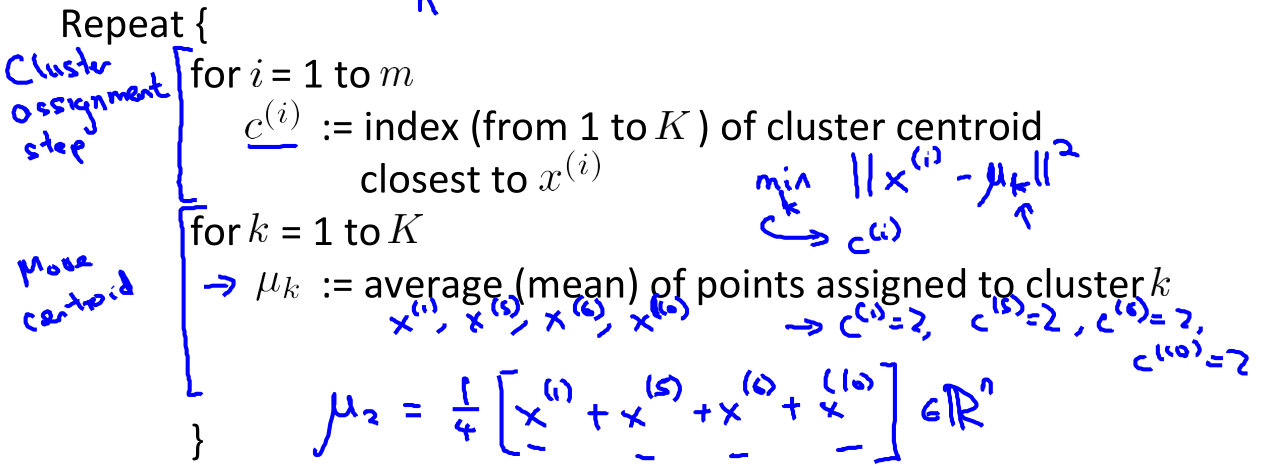• K-means for non-separated clusters

#### 24.3 Optimization Objective

• Notations:

• $c^{(i)}$ = index of cluster ($1, 2, \dots, K$) to which example $x^{(i)}$ is currently assigned
• $c^{(i)} = k \in {1, 2, \dots, K}$
• $i \in {1, 2, \dots, m}$
• $\mu_k$ = cluster centroid k ($\mu_k \in \Bbb{R}^n$)
• $\mu_{c^{(i)}}$ = cluster centroid of cluster to which example $x^{(i)}$ has been assigned
• Optimization objective

• If we consider the K-means algorithm

• The cluster assigned step is minimizing $J(\cdot)$ with respect to $c^{(i)}$
• i.e. find the centroid closet to each example
• Doesn’t change the centroids themselves
• The move centroid step
• This step is choosing the values of $\mu$ which minimizes $J(\cdot)$ with respect to $\mu_k$
• So, we’re partitioning the algorithm into two parts:
• First part minimizes the $c$ variables
• Second part minimizes the $J$ variables

#### 24.4 Random Initialization

• Have number of centroids set to less than number of examples ($K < m$)
• Randomly pick $K$ training examples
• Set $\mu_1$ up to $\mu_K$ to these examples values
• K-means can converge to different solutions depending on the initialization setup
• Risk of local optimum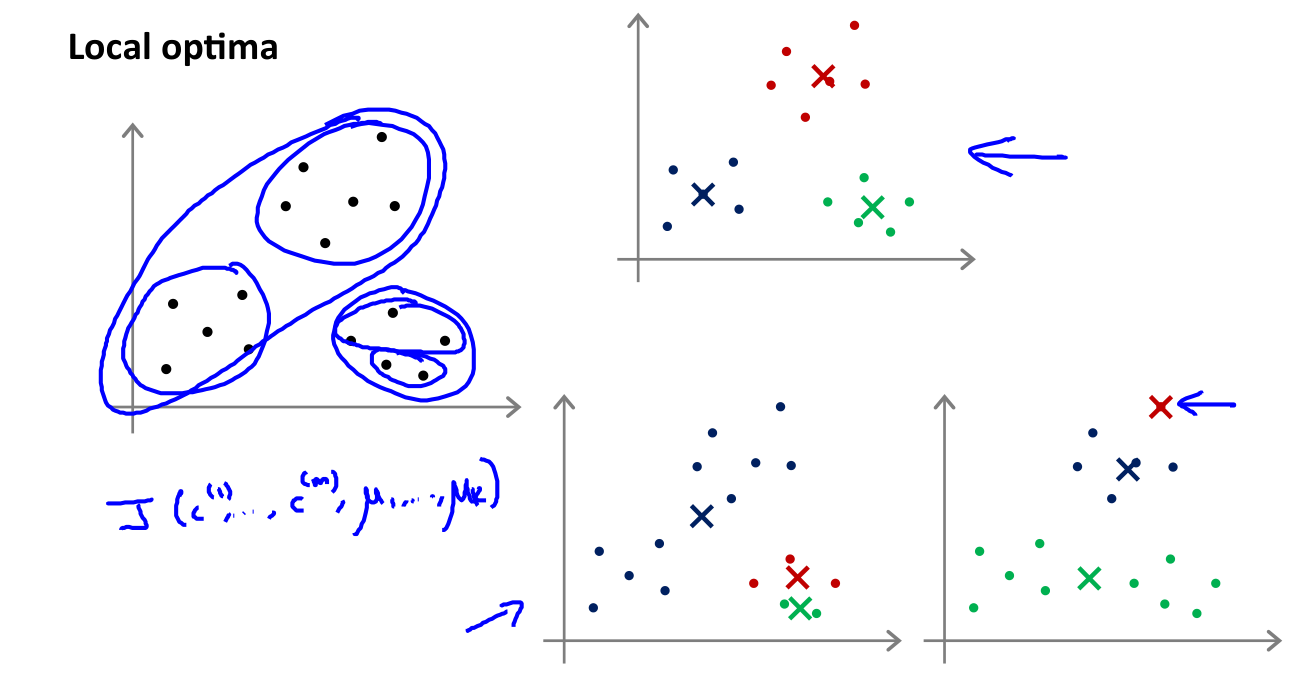• We can do multiple random initializations to see if we get the same result - many same result are likely to indicate a global optimum

#### 24.5 Choosing the Number of Clusters

• What is right value of $K$ ?
• Elbow method
• Vary $K$ and compute cost function at a range of $K$ values
• As $K$ increases, $J(\cdot)$ minimum value should decrease (i.e. you decrease the granularity so centroids can better optimize)
• Plot $K$ vs. $J(\cdot)$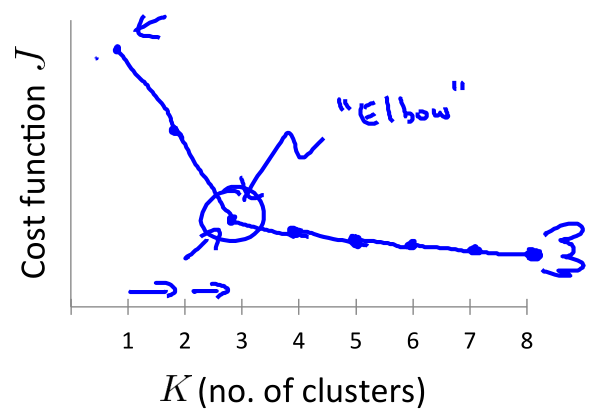• Chose the “elbow” number of clusters (if you get a nice plot this is a reasonable way of choosing $K$)
• Risks: normally you don’t get a a nice line → no clear elbow on curve
• Another method for choosing $K$
• Sometimes, you’re, running K-­means to get clusters to use for some later/downstream purpose. Evaluate K­‐means based on a metric for how well it performs for that later purpose.

## 25 Motivation

#### 25.1 Motivation I: Data Compression

• A second type of unsupervised learning algorithm: dimensionality reduction
• Data compression
• Speeds up algorithms
• Reduces space used by data for them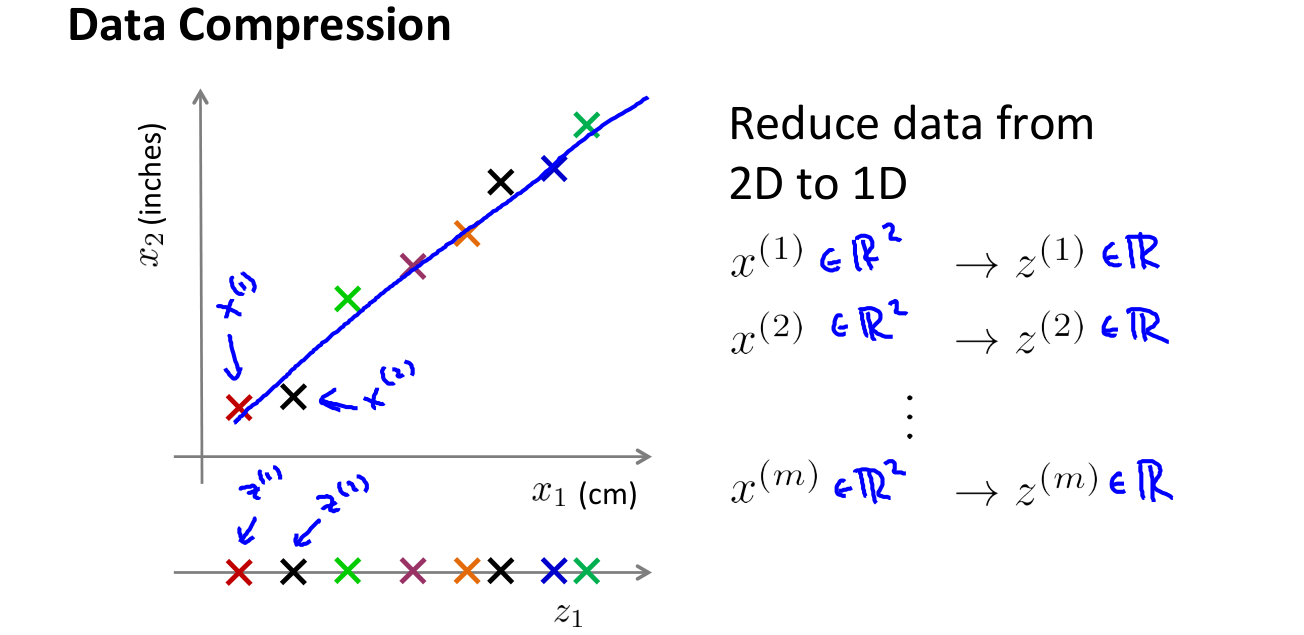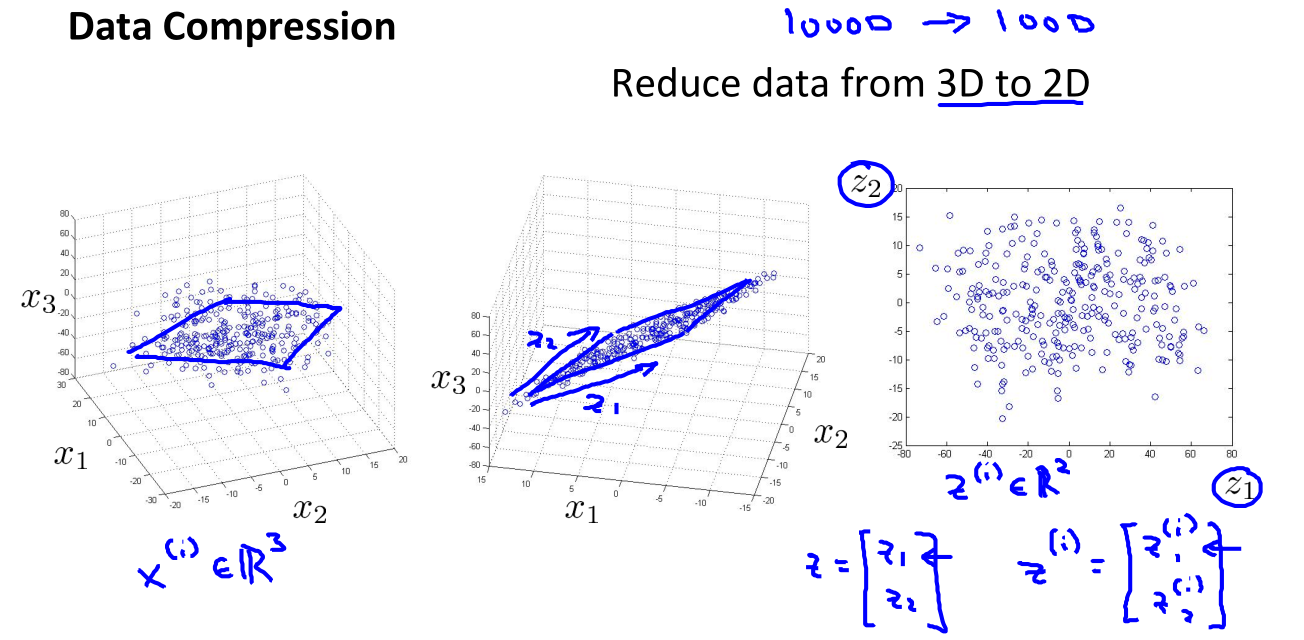#### 25.2 Motivation II: Visualization

• It’s hard to visualize highly dimensional data
• Dimensionality reduction can improve how we display information in a tractable manner for human consumption
• Why do we care?
• Often helps to develop algorithms if we can understand our data better
• Dimensionality reduction helps us do this, see data in a helpful manner
• Good for explaining something to someone if you can “show” it in the data

## 26 Principal Component Analysis

#### 26.1 Principal Component Analysis Problem Formulation

• For the problem of dimensionality reduction the most commonly used algorithm is PCA

• Say we have a 2D data set which we wish to reduce to 1D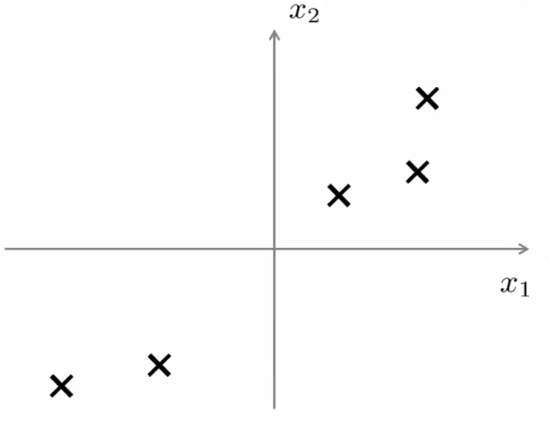• In other words, find a single line onto which to project this data. How do we determine this line?

• The distance between each point and the projected version should be small (blue lines below are short)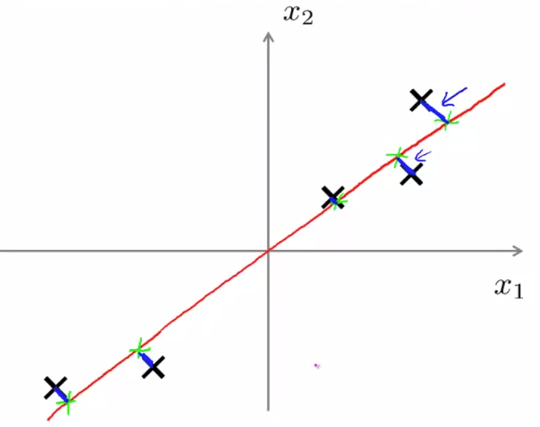• PCA tries to find a lower dimensional surface so the sum of squares onto that surface is minimized

• The blue lines are sometimes called the projection error

• PCA tries to find the surface (a straight line in this case) which has the minimum projection error
• As an aside, you should normally do mean normalization and feature scaling on your data before PCA

• A more formal description is

• For 2D-1D, we must find a vector $u^{(1)}$, which is of some dimensionality
• Onto which you can project the data so as to minimize the projection error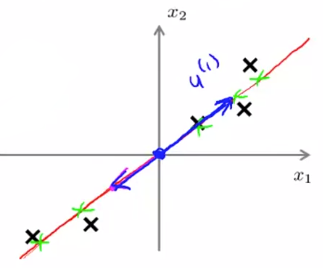• $u^{(1)}$ can be positive or negative ($-u^{(1)}$) which makes no difference
• Each of the vectors define the same red line
• In the more general case, To reduce from $n$-D to $k$-D we

• Find $k$ vectors ($u^{(1)} , u^{(2)}, \dots, u^{(k)}$) onto which to project the data to minimize the projection error
• So lots of vectors onto which we project the data
• Find a set of vectors which we project the data onto the linear subspace spanned by that set of vectors
• We can define a point in a plane with $k$ vectors
• e.g. 3D → 2D
• Find pair of vectors which define a 2D plane (surface) onto which you’re going to project your data
• Much like the “shallow box” example in compression, we’re trying to create the shallowest box possible (by defining two of it’s three dimensions, so the box’ depth is minimized)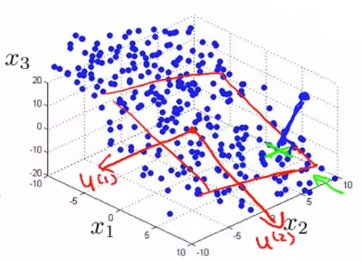• PCA is NOT linear regression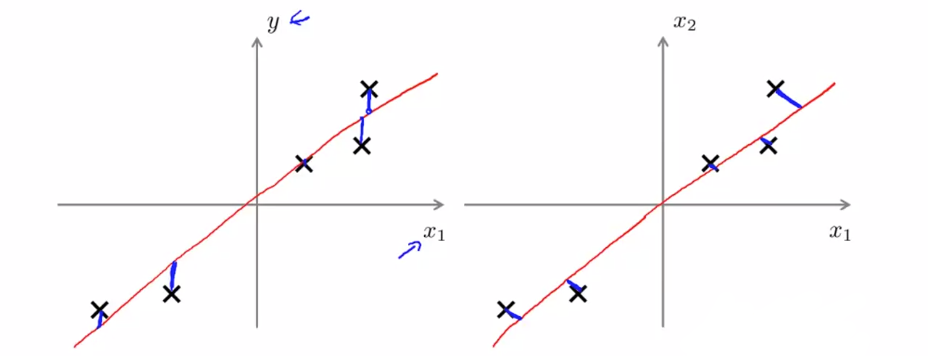• For linear regression, fitting a straight line to minimize the straight line between a point and a squared line
• Note: VERTICAL distance between point
• For PCA minimizing the magnitude of the shortest orthogonal distance
• Gives very different effects
• More generally
• With linear regression we’re trying to predict “$y$”
• With PCA there is no “$y$” - instead we have a list of features and all features are treated equally
• If we have 3D dimensional data 3D->2D
• Have 3 features treated symmetrically

#### 26.2 Principal Component Analysis Algorithm

• Before applying PCA must do data preprocessing

• Mean normalization
• Feature scaling (depending on data)
• With preprocessing done, PCA finds the lower dimensional sub-space which minimizes the sum of the square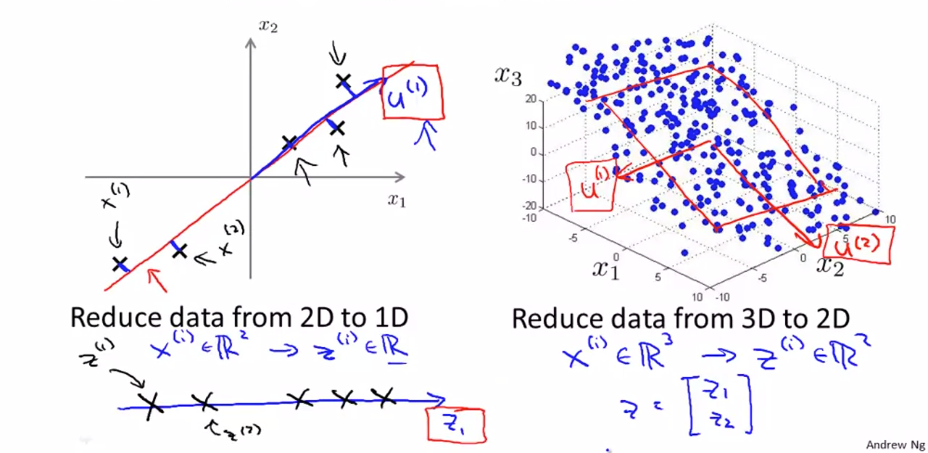• Need to compute two things: $\mu$ vectors & $z$ vectors
• Algorithm description

• Reducing data from $n$-dimensional to $k$-dimensional

• Compute the covariance matrix: $\Sigma = \frac{1}{m}\sum_{i=1}^n \left(x^{(i)} \right)\left(x^{(i)} \right)^T$

• $\Sigma$: This is commonly denoted as Σ (Greek upper case sigma) - NOT summation symbol
• $\Sigma = \frac{1}{m} x^Tx$
• $\Sigma$ is an $n \times n$ matrix
• $x^{(i)}$ is an $n \times 1$ matrix
• Compute eigenvectors of matrix $\Sigma$: [U, S, V] = svd(sigma)

• svd = singular value decomposition
• $U$, $S$ and $V$ are matrices

• $U$ matrix is also an $n \times n$ matrix

• Turns out the columns of $U$ are the $u$ vectors we want

• So to reduce a system from $n$-D to $k$-D: just take the first $k$ vectors from $U$: $% $

• Next we need to find some way to change $x$ (which is $n$ dimensional) to $z$ (which is $k$ dimensional) - reduce the dimensionality

• Take first $k$ columns of the $u$ matrix and stack in columns: $% $

• $z = U_{\text{reduce}}^T * x=[k \times n] * [n \times 1] = [k \times 1]$

• Exactly the same as with supervised learning except we’re now doing it with unlabeled data

• So in summary

• Preprocessing
• Calculate $\Sigma$ (covariance matrix)
• Calculate eigenvectors with svd
• Take $k$ vectors from $U$, i.e. $U_{\text{reduce}} = U(:,1:k)$
• Calculate $z$ ($z =U_{\text{reduce}}^T * x$)

## 27 Applying PCA

#### 27.1 Reconstruction from compressed Representation

• Earlier spoke about PCA as a compression algorithm

• If this is the case, is there a way to decompress the data from low dimensionality back to a higher dimensionality format?
• Reconstruction

• Say we have an example as follows: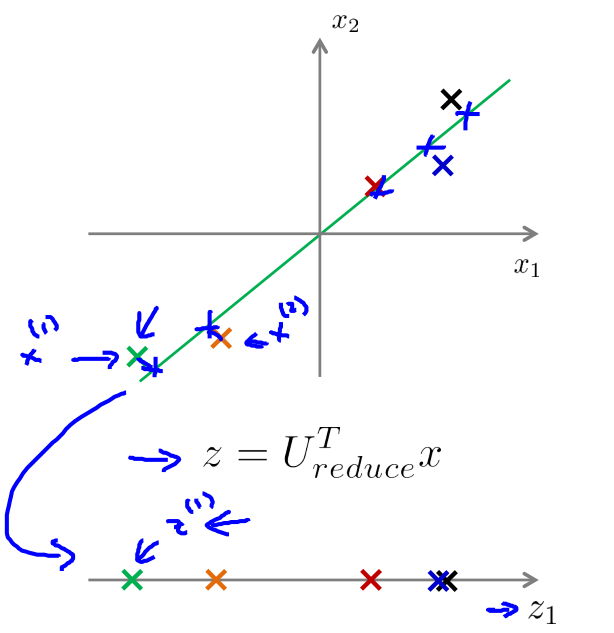• We have our examples ($x^{(1)}$, $x^{(2)}$ etc.)

• Project onto $z$-surface

• Given a point $z^{(1)}$, how can we go back to the 2D space?

• Considering

• $z = U_{\text{reduce}}^T * x$
• To go in the opposite direction we must do

• $x_{\text{approx}} = U_{\text{reduce}} * z\,(=[n\times k] * [k * 1] = [n \times 1])$
• So this creates the following representation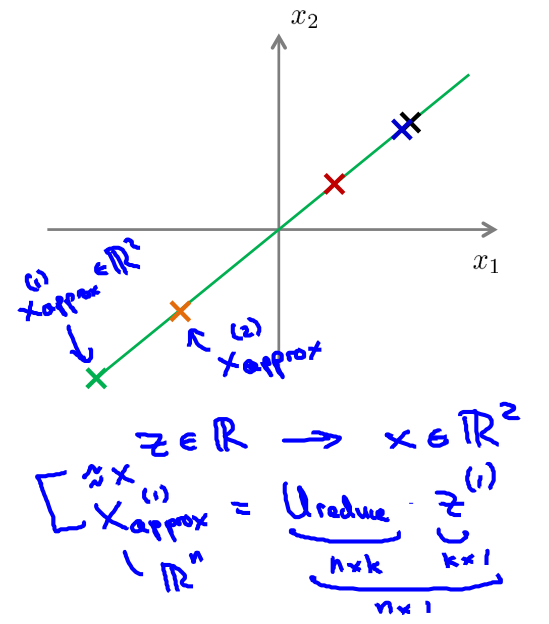• We lose some of the information (i.e. everything is now perfectly on that line) but it is now projected into 2D space

#### 27.2 Choosing the Number of Principal Components

• PCA tries to minimize average squared projection error: $\frac{1}{m} \sum_{i=1}^m \| x^{(i)} - x^{(i)}_{\text{approx}} \|^2$

• Total variation in data can be defined as the average over data saying how far are the training examples from the origin: $\frac{1}{m} \sum_{i=1}^m \| x^{(i)} \|^2$

• Typically, choose $k$ to be smallest value so that $\frac{\frac{1}{m} \sum_{i=1}^m \| x^{(i)} - x^{(i)}_{\text{approx}} \|^2}{\frac{1}{m} \sum_{i=1}^m \| x^{(i)} \|^2} \le 0.01 \, (=1\%)$

• means “99% of variance is retained”
• Algorithms:

• Try PCA with $k = 1$

• Compute $U_{\text{reduce}}, z^{(1)}, \dots,z^{(m)}, x^{(1)}_{\text{approx}}, \dots,x^{(m)}_{\text{approx}}$

• check if the ratio mentioned above is less than (or equal to) 0.01?

• if not, try $k = 2, \dots$
• Easier way: [U, S, V] = svd(Sigma)

• $S$ is an $n \times n$ diagonal matrix: $% $

• And $\frac{\frac{1}{m} \sum_{i=1}^m \| x^{(i)} - x^{(i)}_{\text{approx}} \|^2}{\frac{1}{m} \sum_{i=1}^m \| x^{(i)} \|^2} = 1 - \frac{\sum_{i=1}^kS_{ii}}{\sum_{i=1}^nS_{ii}}$

• Pick smallest value of $k$ which: $\frac{\sum_{i=1}^kS_{ii}}{\sum_{i=1}^nS_{ii}} \ge 0.99$

#### 27.3 Advice for Applying PCA

• Speeding up supervised learning algorithm
• Say you have a supervised learning problem
• Input $x$ and $y$
• $x$ is a 10,000 dimensional feature vector
• e.g. $100 \times100$ images = 10,000 pixels
• Such a huge feature vector will make the algorithm slow
• With PCA we can reduce the dimensionality and make it tractable
• How
• 1) Extract $x$
• So we now have an unlabeled training set
• 2) Apply PCA to $x$ vectors
• So we now have a reduced dimensional feature vector $z$
• 3) This gives you a new training set
• Each vector can be re-associated with the label
• 4) Take the reduced dimensionality data set and feed to a learning algorithm
• Use $y$ as labels and $z$ as feature vector
• 5) if you have a new example map from higher dimensionality vector to lower dimensionality vector, then feed into learning a algorithm
• PCA maps one vector to a lower dimensionality vector
• $x \rightarrow z$
• Defined by PCA only on the training set
• The mapping computes a set of parameters
• Feature scaling values
• $U_{\text{reduce}}$
• Parameter learned by PCA
• Should be obtained only by determining PCA on your training set
• So we use those learned parameters for our
• Cross validation set
• Test set
• Applications of PCA
• Compression
• Reduce memory/disk needed to store data
• Speed up learning algorithm
• Visualization
• Typically chose $k =2$ or $k = 3$
• Because we can plot these values!
• A bad use of PCA: Use it to prevent over-fitting
• Reasoning
• If we have $x^i$ we have $n$ features, $z^i$ has $k$ features which can be lower
• If we only have k features then maybe we’re less likely to over fit…
• This doesn’t work
• Might work OK, but not a good way to address over fitting
• Better to use regularization
• PCA throws away some data without knowing what the values it’s losing
• Probably OK if you’re keeping most of the data
• But if you’re throwing away some crucial data bad
• So you have to go to like 95-99% variance retained
• So here regularization will give you AT LEAST as good a way to solve over fitting
• A second PCA myth
• Used for compression or visualization - good
• Sometimes used to
• Design ML system with PCA from the outset
• But, what if you did the whole thing without PCA? (try it)
• See how a system performs without PCA
• When to use PCA: ONLY if you have a reason to believe PCA will help should you then add PCA
• PCA is easy enough to add on as a processing step
• Try without first!

## Ex7: K-Means Clustering and PCA👨‍💻

See this exercise on Coursera-MachineLearning-Python/ex7/ex7.ipynb

Thistledown